Next: The Complete Algorithm Up: Generating the Edit Previous: Outline of Algorithm

## Aligning Children

The align phase of the edit script algorithm presents an interesting problem. Suppose we detect that for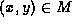, the children of x and y are misaligned. In general, there is more than one sequence of moves that will align the children. For instance, in Figure 7 there are at least two ways to align the children of nodes 1 and 11. The first consists of moving nodes 2 and 4 to the right of node 6, and the second consists of moving nodes 3, 5, and 6 to the left of node 2. Both yield the same final configuration, but the first one is better since it involves fewer moves.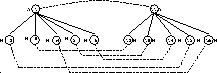Figure 7: A matching with misaligned nodes

To ensure that the edit script generated by the algorithm is of minimum cost, we must find the shortest sequence of moves to align the children of x and y. Our algorithm for finding the shortest sequence of moves is based on the notion of a longest common subsequence, described next.

Given a sequence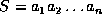, a sequence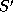is a subsequence of S if it can be obtained by deleting zero or more elements from S. That is,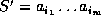where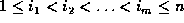. Given two sequences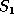and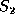, a longest common subsequence (LCS) ofand, denoted by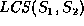, is a sequence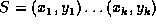of pairs of elements such that (1)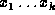is a subsequence of; (2)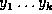is a subsequence of; (3) for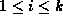,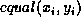is true for some predefined equality function equal; and (4) there is no sequencethat satisfies conditions 1, 2, and 3 and is longer than S. The length of an LCS ofandis denoted by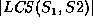.

We use an algorithm due to Myers [Mye86] that computes an LCS of two sequences in time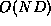, where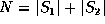and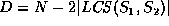. We treat Myers' LCS algorithm as having three inputs: the two sequencesandto be compared, and an equality function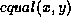used to compare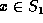and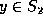for equality. That is, we treat it as the procedure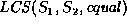.

The solution to the alignment problem is now straightforward. Compute an LCS S of the matched children of nodes x and y, using the equality function equal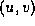that is true if and only if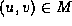. Leave the children of x that are in S fixed, and move the remaining matched children of x to the correct positions relative to the already aligned children. In Figure 7, the LCS is 3,5,6 (matching the sequence 12,13,14). The moves generated are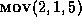and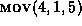. In [CRGMW95], we show that our LCS-based strategy always leads to the minimum number of moves.Next: The Complete Algorithm Up: Generating the Edit Previous: Outline of Algorithm

Sudarshan S. Chawathe
Wed Jun 19 08:22:34 PDT 1996